## Chapter 4Boundary Conditions

“Sprawling on the fringes of the city, in geometric order, an insulated border.”

Rush, Subdivisions (1982).

Chapter 3 described:

• the computational mesh consisting of cells of any irregular polyhedral shape with mesh properties for the ﬁnite volume method;
• discretisation of terms in equations to construct a matrix equation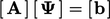for solution variable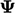;
• discretisation schemes for optimal accuracy, boundedness and convergence, using limiting and correction;
• the time derivative, Courant number and time step size.

At the boundary of the mesh, constraints must be applied to the solution variable, known as boundary conditions. Setting boundary conditions is challenging because:

• they need to reﬂect the physical conditions of the case being simulated;
• they need to make the case well posed, i.e. provide unique, stable, physical solutions to the equations;
• they must be compatible across sets of multiple equations, in particular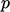and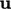.

The mesh boundary is split into regions known as patches, on which diﬀerent boundary conditions are applied. The choice of boundary condition generally depends on ﬂow direction at a patch, whether the patch corresponds to a solid wall, etc.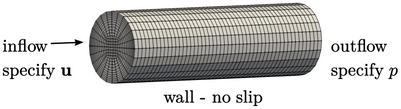There are basic forms of boundary condition which specify the value, normal gradient, etc. of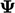at the boundary. They are applied through modiﬁcations to the matrix coeﬃcients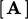and source vector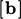using mesh data of the faces and cells adjacent to the boundary.

The opening topics of this chapter describe the mesh data and the numerical methods required by the basic forms of boundary condition.

More specialised boundary conditions can be derived from the underlying forms which correspond to diﬀerent physical conditions. Some derived conditions, that are often used for particular boundary conﬁgurations, are introduced in this chapter.

Otherwise, the number of possible derived conditions is almost unlimited due to the range of potential physical conditions that can be encountered in ﬂuid ﬂow problems. It is left to the producers of CFD software to document those conditions, when it is important that the user identiﬁes the basic underlying type of the condition, e.g. ﬁxed value or gradient.

Notes on CFD: General Principles - Chapter 4 Boundary Conditions